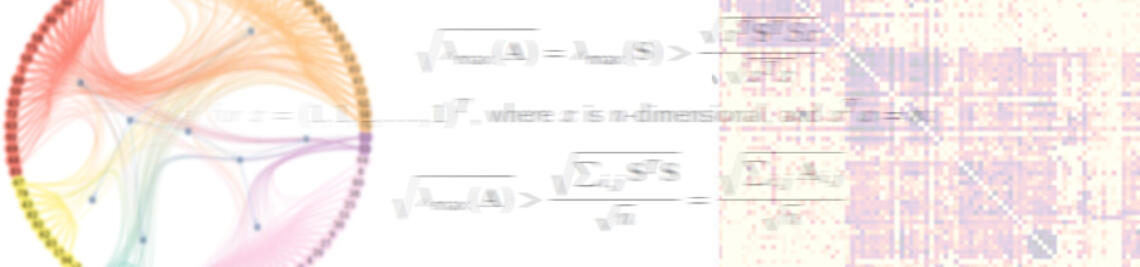•# De Bruijn Graph Neural Networks

09/29/2022

We have just released a new preprint on De Bruijn Graph Neural Networks, a new approach to time-aware graph learning that leverages the causal topology of time-stamped network data.

We introduce De Bruijn Graph Neural Networks (DBGNNs), a novel time-aware graph neural network architecture for time-resolved data on dynamic graphs. Our approach accounts for temporal-topological patterns that unfold in the causal topology of dynamic graphs, which is determined by causal walks, i.e. temporally ordered sequences of links by which nodes can influence each other over time. Our architecture builds on multiple layers of higher-order De Bruijn graphs, an iterative line graph construction where nodes in a De Bruijn graph of order k represent walks of length k-1, while edges represent walks of length k.

In our preprint, we develop a graph neural network architecture that utilizes De Bruijn graphs to implement a message passing scheme that follows a non-Markovian dynamics, which enables us to learn patterns in the causal topology of a dynamic graph. Addressing the issue that De Bruijn graphs with different orders k can be used to model the same data set, we further apply statistical model selection to determine the optimal graph topology to be used for message passing. An evaluation in synthetic and empirical data sets suggests that DBGNNs can leverage temporal patterns in dynamic graphs, which substantially improves the performance in a supervised node classification task.

The preprint is available on arXiv